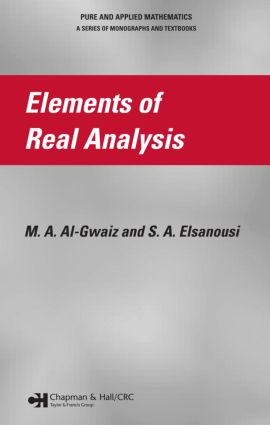Elements of Real Analysis

1st Edition

Chapman and Hall/CRC

436 pages | 60 B/W Illus.

Purchasing Options:\$ = USD
Hardback: 9781584886617
pub: 2006-08-21
SAVE ~\$27.00
\$135.00
\$108.00
x
eBook (VitalSource) : 9780429149283
pub: 2006-08-21
from \$67.50

FREE Standard Shipping!

Description

Focusing on one of the main pillars of mathematics, Elements of Real Analysis provides a solid foundation in analysis, stressing the importance of two elements. The first building block comprises analytical skills and structures needed for handling the basic notions of limits and continuity in a simple concrete setting while the second component involves conducting analysis in higher dimensions and more abstract spaces.

Largely self-contained, the book begins with the fundamental axioms of the real number system and gradually develops the core of real analysis. The first few chapters present the essentials needed for analysis, including the concepts of sets, relations, and functions. The following chapters cover the theory of calculus on the real line, exploring limits, convergence tests, several functions such as monotonic and continuous, power series, and theorems like mean value, Taylor's, and Darboux's. The final chapters focus on more advanced theory, in particular, the Lebesgue theory of measure and integration.

Requiring only basic knowledge of elementary calculus, this textbook presents the necessary material for a first course in real analysis. Developed by experts who teach such courses, it is ideal for undergraduate students in mathematics and related disciplines, such as engineering, statistics, computer science, and physics, to understand the foundations of real analysis.

PREFACE

PRELIMINARIES

Sets

Functions

REAL NUMBERS

Field Axioms

Order Axioms

Natural Numbers, Integers, Rational Numbers

Completeness Axiom

Decimal Representation of Real Numbers

Countable Sets

SEQUENCES

Sequences and Convergence

Properties of Convergent Sequences

Monotonic Sequences

The Cauchy Criterion

Subsequences

Upper and Lower Limits

Open and Closed Sets

INFINITE SERIES

Basic Properties

Convergence Tests

LIMIT OF A FUNCTION

Limit of a Function

Basic Theorems

Some Extensions of the Limit

Monotonic Functions

CONTINUITY

Continuous Functions

Combinations of Continuous Functions

Continuity on an Interval

UniformContinuity

Compact Sets and Continuity

DIFFERENTIATION

The Derivative

TheMean Value Theorem

L'Hôpital's Rule

Taylor's Theorem

THE RIEMANN INTEGRAL

Riemann Integrability

Darboux's Theorem and Riemann Sums

Properties of the Integral

The Fundamental Theorem of Calculus

Improper Integrals

SEQUENCES AND SERIES OF FUNCTIONS

Sequences of Functions

Properties of Uniform Convergence

Series of Functions

Power Series

LEBESGUE MEASURE

Classes of Subsets of R

Lebesgue Outer Measure

Lebesgue Measure

Measurable Functions

LEBESGUE INTEGRATION

Definition of the Lebesgue Integral

Properties of the Lebesgue Integral

Lebesgue Integral and Pointwise Convergence

Lebesgue and Riemann Integrals

REFERENCES

NOTATION

INDEX